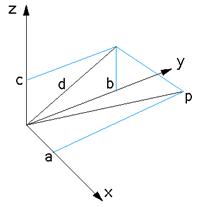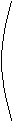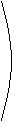# Rotate a pointabout an arbitrary axis(3 dimensions)

Written by Paul Bourke
December 1992, Updated August 2002

Illustrative C code that implements the following algorithm is given here. A closed solution attributed to Ronald Goldman is presented as this C function. A contribution by Bruce Vaughan in the form of a Python script for the SDS/2 design software: PointRotate.py.Rotation of a point in 3 dimensional space by theta about an arbitrary axes defined by a line between two points P1 = (x1,y1,z1) and P2 = (x2,y2,z2) can be achieved by the following steps

(1) translate space so that the rotation axis passes through the origin

(2) rotate space about the x axis so that the rotation axis lies in the xz plane

(3) rotate space about the y axis so that the rotation axis lies along the z axis

(4) perform the desired rotation by theta about the z axis

(5) apply the inverse of step (3)

(6) apply the inverse of step (2)

(7) apply the inverse of step (1)

Note:

• If the rotation axis is already aligned with the z axis then steps 2, 3, 5, and 6 need not be performed.

• In all that follows a right hand coordinate system is assumed and rotations are positive when looking down the rotation axis towards the origin.

• Symbols representing matrices will be shown in bold text.

• The inverse of the rotation matrices below are particularly straightforward since the determinant is unity in each case.

• All rotation angles are considered positive if anticlockwise looking down the rotation axis towards the origin.

Step 1

Translate space so that the rotation axis passes through the origin. This is accomplished by translating space by -P1 (-x1,-y1,-z1). The translation matrix T and the inverse T-1 (required for step 7) are given below

T =1 0 0 -x1 0 1 0 -y1 0 0 1 -z1 0 0 0 1T-1 =1 0 0 x1 0 1 0 y1 0 0 1 z1 0 0 0 1Step 2

Rotate space about the x axis so that the rotation axis lies in the xz plane. Let U = (a,b,c) be the unit vector along the rotation axis. and define d = sqrt(b2 + c2) as the length of the projection onto the yz plane. If d = 0 then the rotation axis is along the x axis and no additional rotation is necessary. Otherwise rotate the rotation axis so that is lies in the xz plane. The rotation angle to achieve this is the angle between the projection of rotation axis in the yz plane and the z axis. This can be calculated from the dot product of the z component of the unit vector U and its yz projection. The sine of the angle is determine by considering the cross product.

 (0,0,c) dot (0,b,c) cos(t) = = c/d c d

 || (0,0,c) cross (0,b,c) || sin(t) = = b/d c d

The rotation matrix Rx and the inverse Rx-1 (required for step 6) are given below

Rx =1 0 0 0 0 c/d -b/d 0 0 b/d c/d 0 0 0 0 1Rx-1 =1 0 0 0 0 c/d b/d 0 0 -b/d c/d 0 0 0 0 1Step 3

Rotate space about the y axis so that the rotation axis lies along the positive z axis. Using the appropriate dot and cross product relationships as before the cosine of the angle is d, the sine of the angle is a. The rotation matrix about the y axis Ry and the inverse Ry-1 (required for step 5) are given below.

Ry =d 0 -a 0 0 1 0 0 a 0 d 0 0 0 0 1Ry-1 =d 0 a 0 0 1 0 0 -a 0 d 0 0 0 0 1Step 4

Rotation about the z axis by t (theta) is Rz and is simply

Rz =cos(t) -sin(t) 0 0 sin(t) cos(t) 0 0 0 0 1 0 0 0 0 1The complete transformation to rotate a point (x,y,z) about the rotation axis to a new point (x`,y`,z`) is as follows, the forward transforms followed by the reverse transforms.x' y' z' 1= T-1 Rx-1 Ry-1 Rz Ry Rx Tx y z 1### Using quaternions

To rotate a 3D vector "p" by angle theta about a (unit) axis "r" one forms the quaternion

Q1 = (0,px,py,pz)

and the rotation quaternion

Q2 = (cos(theta/2), rx sin(theta/2), ry sin(theta/2), rz sin(theta/2)).

The rotated vector is the last three components of the quaternion

Q3 = Q2 Q1 Q2*

It is easy to see that rotation in the opposite direction (-theta) can be achieved by reversing the order of the multiplication.

Q3 = Q2* Q1 Q2

Note also that the quaternion Q2 is of unit magnitude.